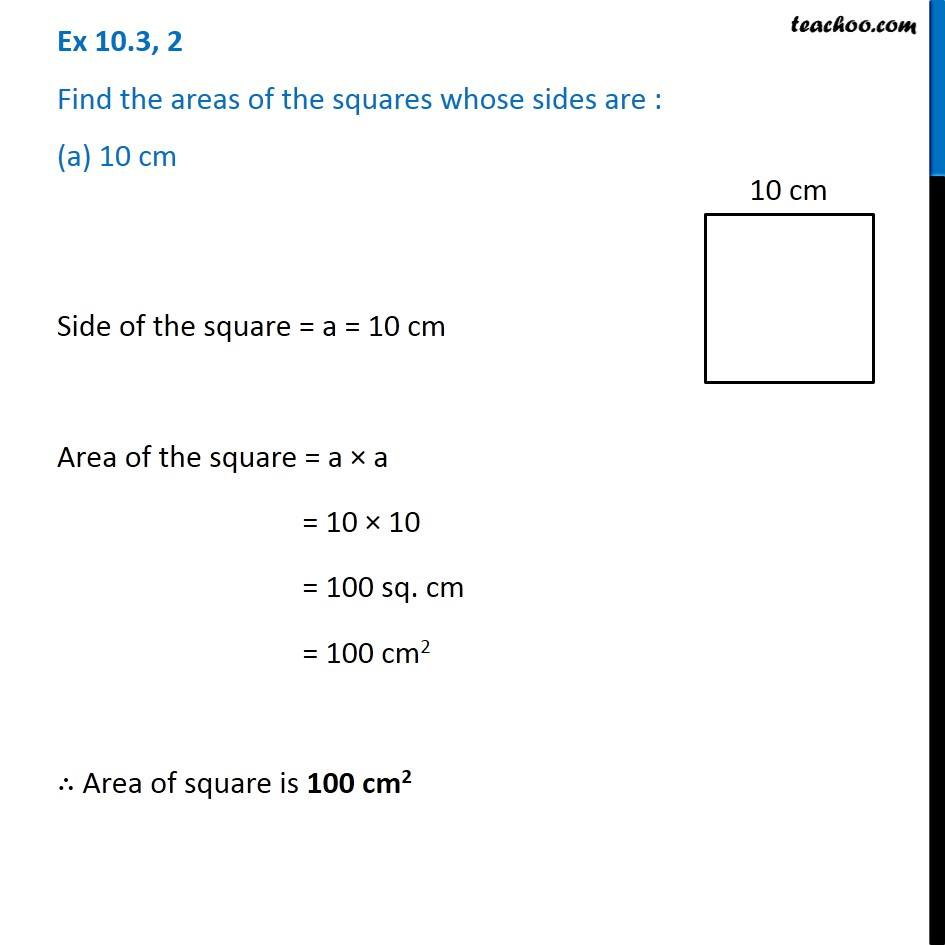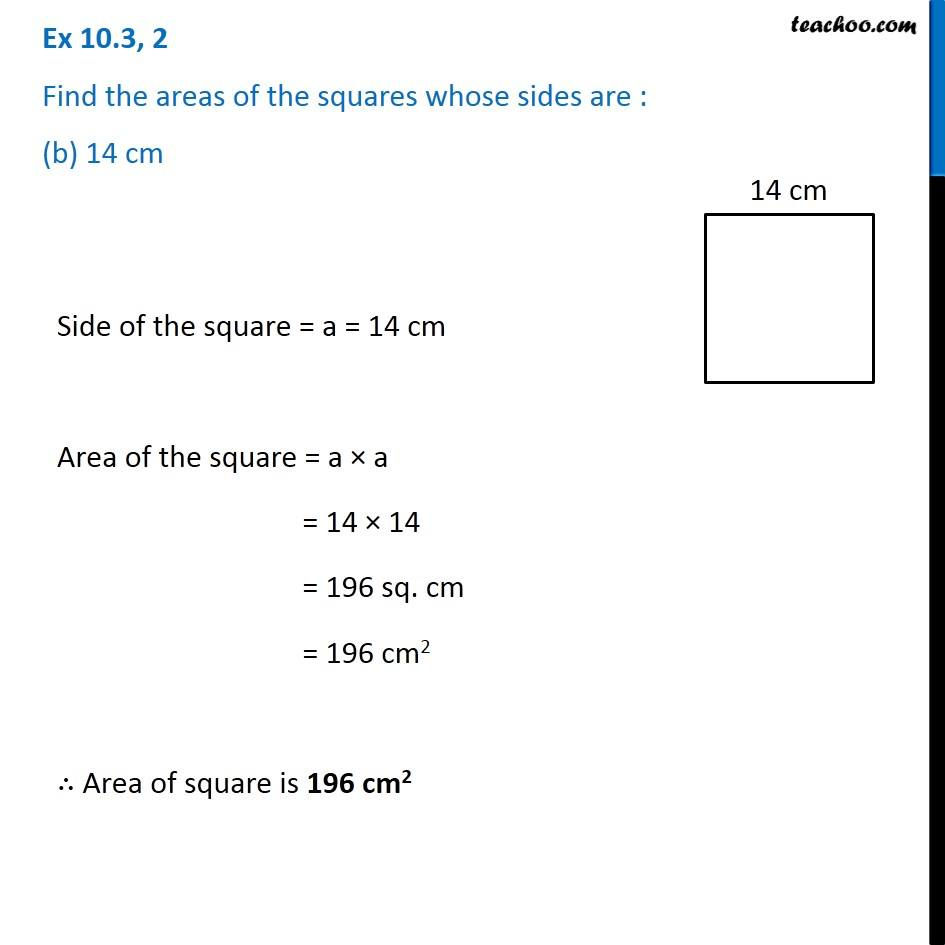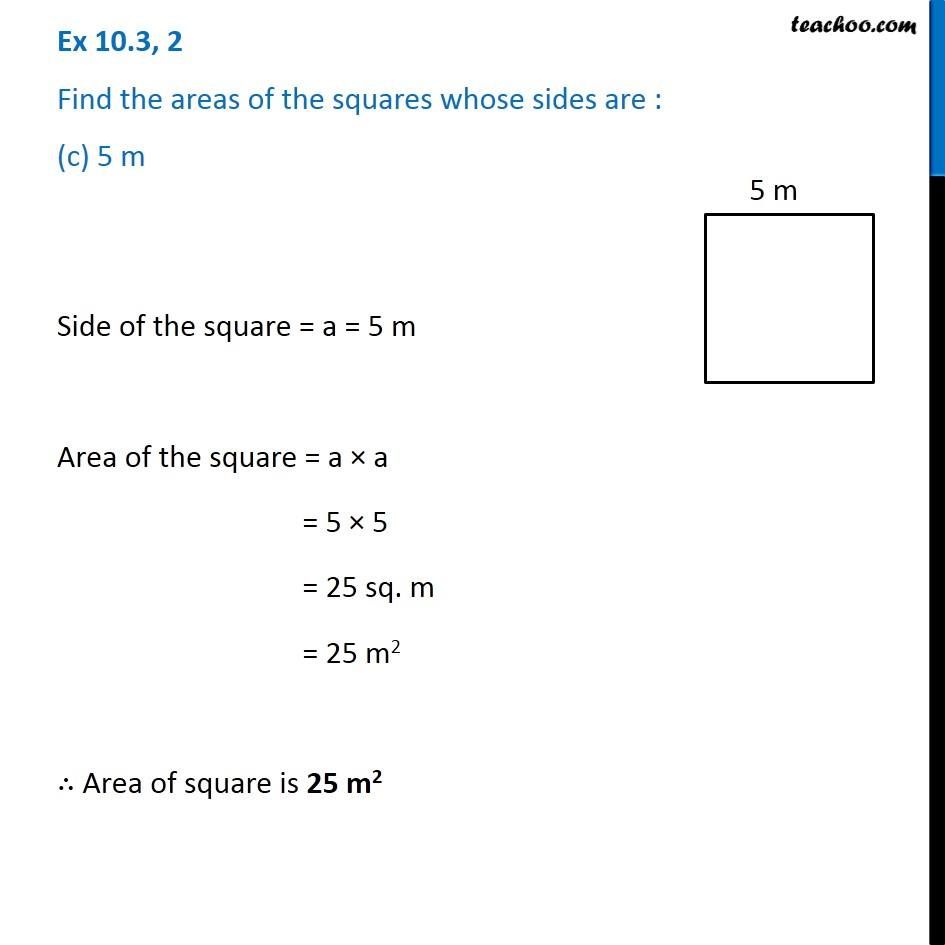Subscribe to our Youtube Channel - https://you.tube/teachoo

1. Chapter 10 Class 6 Mensuration
2. Serial order wise
3. Ex 10.3

Transcript

Ex 10.3, 2 Find the areas of the squares whose sides are : (a) 10 cm Side of the square = a = 10 cm Area of the square = a × a = 10 × 10 = 100 sq. cm = 100 cm2 ∴ Area of square is 100 cm2 Ex 10.3, 2 Find the areas of the squares whose sides are : (b) 14 cm Side of the square = a = 14 cm Area of the square = a × a = 14 × 14 = 196 sq. cm = 196 cm2 ∴ Area of square is 196 "cm2" Ex 10.3, 2 Find the areas of the squares whose sides are : (c) 5 m Side of the square = a = 5 m Area of the square = a × a = 5 × 5 = 25 sq. m = 25 "m2" ∴ Area of square is 25 m2

Ex 10.3

Chapter 10 Class 6 Mensuration
Serial order wise